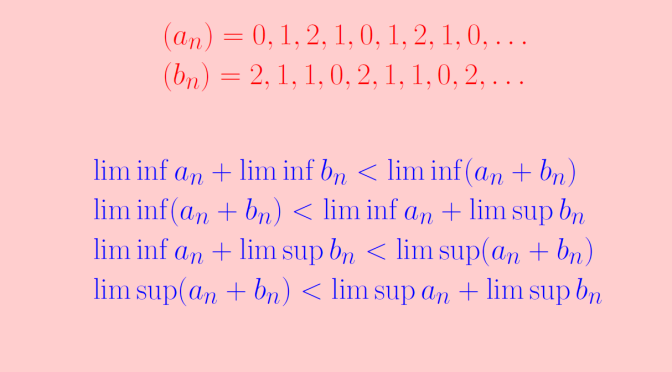# Playing with liminf and limsup

Let’s consider real sequences $$(a_n)_{n \in \mathbb N}$$ and $$(b_n)_{n \in \mathbb N}$$. We look at inequalities involving limit superior and limit inferior of those sequences. Following inequalities hold:
\begin{aligned} & \liminf a_n + \liminf b_n \le \liminf (a_n+b_n)\\ & \liminf (a_n+b_n) \le \liminf a_n + \limsup b_n\\ & \liminf a_n + \limsup b_n \le \limsup (a_n+b_n)\\ & \limsup (a_n+b_n) \le \limsup a_n + \limsup b_n \end{aligned} Let’s prove for example the first inequality, reminding first that $\liminf\limits_{n \to \infty} a_n = \lim\limits_{n \to \infty} \left(\inf\limits_{m \ge n} a_m \right).$ For $$n \in \mathbb N$$, we have for all $$m \ge n$$ $\inf\limits_{k \ge n} a_k + \inf\limits_{k \ge n} b_k \le a_m + b_m$ hence $\inf\limits_{k \ge n} a_k + \inf\limits_{k \ge n} b_k \le \inf\limits_{k \ge n} \left(a_k+b_k \right)$ As the sequences $$(\inf\limits_{k \ge n} a_k)_{n \in \mathbb N}$$ and $$(\inf\limits_{k \ge n} b_k)_{n \in \mathbb N}$$ are non-increasing we get for all $$n \in \mathbb N$$, $\liminf a_n + \liminf b_n \le \inf\limits_{m \ge n} \left(a_m+b_m \right)$ which leads finally to the desired inequality $\liminf a_n + \liminf b_n \le \liminf (a_n+b_n).$

In fact, all inequalities above can be strict. For example, take $$a_n = (-1)^n$$ and $$b_n = -(-1)^n$$ we have for all $$n \in \mathbb N$$: $$a_n+b_n=0$$, $$\liminf a_n = \liminf b_n =-1$$. Hence $$\liminf a_n + \liminf b_n = -2$$ while $$\liminf a_n + b_n = 0$$. We can even find sequences $$(a_n)_{n \in \mathbb N}$$ and $$(b_n)_{n \in \mathbb N}$$ such that the four inequalities are simultaneously strict. For this, consider the sequences \begin{aligned} & (a_n) = 0, 1, 2, 1, 0, 1, 2, 1, 0, \dots \text{ with } \liminf a_n=0, \, \limsup a_n=2\\ & (b_n) = 2, 1, 1, 0, 2, 1, 1, 0, 2, \dots \text{ with } \liminf b_n=0, \, \limsup b_n=2 \end{aligned} We have \begin{aligned} & (a_n + b_n) = 2, 2, 3, 1, 2, 2, 3, 1, 2, \dots \\ &\text{and } \liminf (a_n+b_n)=1, \, \limsup (a_n+b_n)=3 \end{aligned} which leads to
\begin{aligned} & \liminf a_n + \liminf b_n &= 0\\ & \liminf (a_n+b_n) &= 1\\ & \liminf a_n + \limsup b_n &= 2\\ & \limsup (a_n+b_n) &= 3\\ & \limsup a_n + \limsup b_n &= 4 \end{aligned}

Other interesting topic is that the inequality $\liminf a_n < \limsup b_n$ can also hold while for all $$n \in \mathbb N$$ $$a_n > b_n$$. This is the case for the sequences \begin{aligned} & a_n = \begin{cases} 2 & \text{if } n \text{ is odd}\\ 0 & \text{if } n \text{ is even} \end{cases}\\ & b_n = \begin{cases} 1 & \text{if } n \text{ is odd}\\ -1 & \text{if } n \text{ is even} \end{cases} \end{aligned}